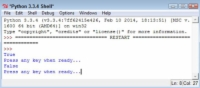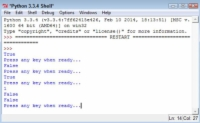How to Create a Queue Based Application in Python - dummies

A queue works differently than a stack in Python. Think of any line you’ve ever stood in: You go to the back of the line, and when you reach the front of the line you get to do whatever you stood in the line to do. A queue is often used for task scheduling and to maintain program flow — just as it is in the real world.

The following steps help you create a queue-based application.

1Open a Python File window.

You see an editor in which you can type the example code.

2Type the following code into the window — pressing Enter after each line:

import queue
MyQueue = queue.Queue(3)
print(MyQueue.empty())
input("Press any key when ready...")
MyQueue.put(1)
MyQueue.put(2)
print(MyQueue.full())
input("Press any key when ready...")
MyQueue.put(3)
print(MyQueue.full())
input("Press any key when ready...")
print(MyQueue.get())
print(MyQueue.empty())
print(MyQueue.full())
input("Press any key when ready...")
print(MyQueue.get())
print(MyQueue.get())

To create a queue, you must import the queue module. This module actually contains a number of queue types, but this example uses only the standard FIFO queue.

When a queue is empty, the empty() function returns True. Likewise, when a queue is full, the full() function returns True. By testing the state of empty() and full(), you can determine whether you need to perform additional work with the queue or whether you can add other information to it.

These two functions help you manage a queue. It’s not possible to iterate through a queue using a for loop as you have done with other collection types, so you must monitor empty() and full() instead.

The two functions used to work with data in a queue are put(), which adds new data, and get(), which removes data. A problem with queues is that if you try to put more items into the queue than it can hold, it simply waits until space is available to hold it.

Unless you’re using a multithreaded application (one that uses individual threads of execution to perform more than one task at one time), this state could end up freezing your application.

3Choose Run→Run Module.

You see a Python Shell window open. The application tests the state of the queue. In this case, you see an output of True, which means that the queue is empty.4Press Enter.

The application adds two new values to the queue. In doing so, the queue is no longer empty.

5Press Enter.

The application adds another entry to the queue, which means that the queue is now full because it was set to a size of 3. This means that full() will return True because the queue is now full.6Press Enter.

To free space in the queue, the application gets one of the entries. Whenever an application gets an entry, the get() function returns that entry. Given that 1 was the first value added to the queue, the print() function should return a value of 1. In addition, both empty() and full() should now return False.

7Press Enter.

The application gets the remaining two entries. You see 2 and 3 (in turn) as output.